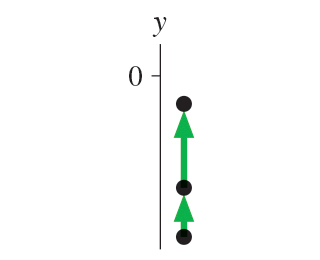Physics Practice Problems Motion Diagrams Practice Problems Solution: Determine the sign (positive, negative, or zero) o...

⚠️Our tutors found the solution shown to be helpful for the problem you're searching for. We don't have the exact solution yet.

# Solution: Determine the sign (positive, negative, or zero) of the position for the particle in .Determine the sign (positive, negative, or zero) of the velocity for the particle in .Determine the sign (positive, negative, or zero) of the acceleration for the particle in .

###### Problem

Determine the sign (positive, negative, or zero) of the position for the particle in.

Determine the sign (positive, negative, or zero) of the velocity for the particle in.

Determine the sign (positive, negative, or zero) of the acceleration for the particle in.

Motion Diagrams

Motion Diagrams

#### Q. Determine the sign (positive, negative, or zero) of the position for the particle in .Determine the sign (positive, negative, or zero) of the vel...

Solved • Mon Oct 29 2018 17:34:30 GMT-0400 (EDT)

Motion Diagrams

#### Q. The figure shows the motion diagram of a drag racer. The camera took one frame every 2 s. You may want to review (Pages 16 - 18). For help with ...

Solved • Mon Oct 29 2018 17:34:29 GMT-0400 (EDT)

Motion Diagrams

#### Q. shows a motion diagram of a car traveling down a street. The camera took one frame every 10 s. A distance scale is provided. You may want to review (...

Solved • Mon Oct 29 2018 17:34:29 GMT-0400 (EDT)

Motion Diagrams

#### Q. Determine the sign (positive, negative, or zero) of the position for the particle in .Determine the sign (positive, negative, or zero) of the vel...

Solved • Mon Oct 29 2018 17:34:28 GMT-0400 (EDT)